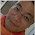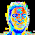## 2015/07/01

### How to calibrate ADXL345 Triple Axis Accelerometer

I recently tested 5 PCs of ADXL345 breakout boards that I bought on Taobao. Sadly, the readouts are so inconsistent and the z-axis readouts are too big to be corrected using the chip's own offset registers located at 0x1E (OSFX), 0x1F (OSFY), and 0x20 (OSFZ).

Below are the readouts of all 5 modules taken with the modules facing down.

Before doing calibration, the first thing is to decide the orientation for taking the measurements. After experimenting with different orientations and observing the readouts, I decided to use the below orientations.

Below is the wiring between ADXL345 and Arduino Uno. Pin 2 of Arduino Uno is connected to GND. When the switch is closed, the program running on Arduino Uno will enter into Calibration Mode. When the switch is open, the program is in Normal Mode.

Program Code

#include <Wire.h>

byte DEVICE_ADDRESS = 0x53; //This address is fixed for the board we use because pin 12 is fixed to GND

byte DATA_FORMAT = 0x31;   //Data Format & Measurement Range Register
byte POWER_CTRL = 0x2D;    //Power Control Register
byte INT_ENABLE = 0x2E;    //Enable Data Ready Interrupt

byte OFSTX = 0x1E;         //X_CALIB
byte OFSTY = 0x1F;         //Y_CALIB
byte OFSTZ = 0x20;         //Z_CALIB

byte DATAX0 = 0x32;        //X-Axis Data 0
byte DATAX1 = 0x33;        //X-Axis Data 1
byte DATAY0 = 0x34;        //Y-Axis Data 0
byte DATAY1 = 0x35;        //Y-Axis Data 1
byte DATAZ0 = 0x36;        //Z-Axis Data 0
byte DATAZ1 = 0x37;        //Z-Axis Data 1

byte values;

int x,y,z;                 //For storing the raw value
double xg, yg, zg;         //For storing the G value

float x_bound = {-9999, -9999, -9999};            //Upper, Lower, Middle
float y_bound = {-9999, -9999, -9999};            //Upper, Lower, Middle
float z_bound = {-9999, -9999, -9999};            //Upper, Lower, Middle

int calibrate = -1;
int CAL_DELAY = 400;      //Calibration Delay - waiting time for user to make response

void setup() {
Wire.begin();    //Initiate the Wire library and join the I2C bus as a master. This is called only once.
Serial.begin(9600);

//Minimum initialization sequance according to the application note.
writeRegister(DEVICE_ADDRESS, DATA_FORMAT, 0x0B); //Put the ADXL345 into Measurement Mode with full resolution (13-bit) and +/-16G range.

pinMode(2, INPUT_PULLUP);     //Pin2 (LOW) -> Calibration Mode, Pin2(HIGH, DEFAULT) -> Normal Mode
}

void loop() {

if (calibration_mode == HIGH) {
//    Serial.println("Normal Mode");
normalMode();

//Converting the raw accelerometer values to g's.

xg = x * 0.0039;
yg = y * 0.0039;
zg = z * 0.0039;

//Display the results

Serial.print(x, DEC);
Serial.print(", ");
Serial.print(y, DEC);
Serial.print(", ");
Serial.print(z, DEC);
Serial.print(" | ");

Serial.print(x_bound);
Serial.print(", ");
Serial.print(x_bound);
Serial.print(", ");
Serial.print(x_bound);
Serial.print(" | ");

Serial.print(y_bound);
Serial.print(", ");
Serial.print(y_bound);
Serial.print(", ");
Serial.print(y_bound);
Serial.print(" | ");

Serial.print(z_bound);
Serial.print(", ");
Serial.print(z_bound);
Serial.print(", ");
Serial.print(z_bound);
Serial.println();

/*
Serial.print((float)xg, 2);
Serial.print("'g, ");
Serial.print((float)yg, 2);
Serial.print("g, ");
Serial.print((float)zg, 2);
Serial.println("g");
*/
}

if (calibration_mode == LOW) {
Serial.println("Calibration Mode");
if ((x_bound == -9999) || (y_bound == -9999) || (z_bound == -9999)) {
Serial.println("Please release pin 2 from logic LOW position");
}
//delay(CAL_DELAY);
calibrationMode();
}
else {
Serial.println("Module already calibrated. Do you want to calibrate again? (0 = No, 1 = Yes) ");
while (calibrate == -1) {
}
if (calibrate == '1') {
calibrationMode();
calibrate = -1;
}
else {
calibrate = -1;
}
}
}
}

void calibrationMode() {

//Get y-axis boundries
//----------------------------------------------------------------------------
Serial.println("Place module to position 1 before count down reaches 0");
for (int i = 25; i >= 0; i--) {
Serial.println(i);
delay(CAL_DELAY);
}
Serial.println();
for (int count = 1; count < 100; count++) {
normalMode();
y_bound = y_bound + y;
}
y_bound = y_bound / 100;
Serial.print("Y Low Bound = ");
Serial.println(y_bound);

Serial.println();
Serial.println("Place module to position 2 before count down reaches 0");
for (int i = 25; i >= 0; i--) {
Serial.println(i);
delay(CAL_DELAY);
}
for (int count = 1; count < 100; count++) {
normalMode();
y_bound = y_bound + y;
}
y_bound = y_bound / 100;
Serial.print("Y High Bound = ");
Serial.println(y_bound);
y_bound = (y_bound + y_bound) / 2;
Serial.print("Y Mid Point = ");
Serial.println(y_bound);

//Get x-axis boundries
//----------------------------------------------------------------------------
Serial.println("Place module to position 3 before count down reaches 0");
for (int i = 25; i >= 0; i--) {
Serial.println(i);
delay(CAL_DELAY);
}
Serial.println();
for (int count = 1; count < 100; count++) {
normalMode();
x_bound = x_bound + x;
}
x_bound = x_bound / 100;
Serial.print("X Low Bound = ");
Serial.println(x_bound);

Serial.println();
Serial.println("Place module to position 4 before count down reaches 0");
for (int i = 25; i >= 0; i--) {
Serial.println(i);
delay(CAL_DELAY);
}
for (int count = 1; count < 100; count++) {
normalMode();
x_bound = x_bound + x;
}
x_bound = x_bound / 100;
Serial.print("X High Bound = ");
Serial.println(x_bound);
x_bound = (x_bound + x_bound) / 2;
Serial.print("X Mid Point = ");
Serial.println(x_bound);

//Get z-axis boundries
//----------------------------------------------------------------------------
Serial.println("Place module to position 5 before count down reaches 0");
for (int i = 25; i >= 0; i--) {
Serial.println(i);
delay(CAL_DELAY);
}
Serial.println();
for (int count = 1; count < 100; count++) {
normalMode();
z_bound = z_bound + z;
}
z_bound = z_bound / 100;
Serial.print("Z Low Bound = ");
Serial.println(z_bound);

Serial.println();
Serial.println("Place module to position 6 before count down reaches 0");
for (int i = 25; i >= 0; i--) {
Serial.println(i);
delay(CAL_DELAY);
}
for (int count = 1; count < 100; count++) {
normalMode();
z_bound = z_bound + z;
}
z_bound = z_bound / 100;
Serial.print("Z High Bound = ");
Serial.println(z_bound);
z_bound = (z_bound + z_bound) / 2;
Serial.print("Z Mid Point = ");
Serial.println(z_bound);

}

void normalMode() {

//The ADXL345 gives 10-bit or 13-bit acceleration values, but they are stored as bytes (8-bits). To get the full value, two bytes must be combined for each axis.
//The X value is stored in values and values.
x = ((int)values<<8)|(int)values;
//The Y value is stored in values and values.
y = ((int)values<<8)|(int)values;
//The Z value is stored in values and values.
z = ((int)values<<8)|(int)values;

delay(15);
}

void writeRegister(byte device, byte registerAddress, byte value) {
Wire.beginTransmission(device);    //Start transmission to device
Wire.write(value);                 //Send the value to be writen to the register
Wire.endTransmission();            //End transmission
}

Wire.beginTransmission(device);
Wire.endTransmission();

Wire.beginTransmission(device);
Wire.requestFrom(device, numBytes);  //Request 6 bytes from device

int i = 0;
while (Wire.available() && i < numBytes) {        //Device may send less than requested
values[i] = Wire.read();                        //Receive a byte from device and put it into the buffer
i++;
}

Wire.endTransmission();
}

Note,
When using the above program, please refer to the 2nd pic of this post for how to position the module.

Output

Below is the screen output.

Here is the same output with added notes.

The Low and High of each axis are the average of 100 sample values. The Mid of each axis is the average of the Low and High of the axis.

With the available of the Low, High, and Mid readouts of each axis, it's now possible to map the readouts to other systems (i.e. degree, etc.).

Things to do
1. Determine the direction of rotation (tilt forward / backward / left / right),
2. Map and show the raw readouts to degree.

1.My output:

-100, -239, 1611 | -506.40, -206.20, -356.30 | -547.12, -483.75, -515.44 | 1233.88, 974.73, 1104.30
-105, -238, 1613 | -506.40, -206.20, -356.30 | -547.12, -483.75, -515.44 | 1233.88, 974.73, 1104.30
-105, -237, 1612 | -506.40, -206.20, -356.30 | -547.12, -483.75, -515.44 | 1233.88, 974.73, 1104.30
-104, -237, 1613 | -506.40, -206.20, -356.30 | -547.12, -483.75, -515.44 | 1233.88, 974.73, 1104.30
-105, -237, 1611 | -506.40, -206.20, -356.30 | -547.12, -483.75, -515.44 | 1233.88, 974.73, 1104.30
-107, -238, 1613 | -506.40, -206.20, -356.30 | -547.12, -483.75, -515.44 | 1233.88, 974.73, 1104.30

2.I suspect a lot of ADXL345 modules available from Taobao or Alibaba have defective ADXL345 chipset on them (some with defective Z axis, some with defective Y axis, some with defective X axis). That's why there are huge variances in the readouts between modules. I heard that the quality of Bosch's chipset is better but I haven't got the chance to try it. I encourage anyone who has the chance to try out the module that uses Bosch chipset and share the result with us..

3.Same problem with modules from Aliexpress.
It's possible to try your code (it's impossible to cut/paste from the website)??
thanks
Luca

1.4.5.1.O.K. I have sent you the code by e-mail just now..

6.Hi there.
Interesting post. Thanks for that.
You know what would be really cool?
If you put your code in a way that people could select and copy. Would you do it? Please?

Best regards

1.Actually, it's quite easy to copy the code even when the selection and copy features are disabled. :-) All the codes on my blog were published with the intention to share them with the community and to make friend. If you want the code, just drop me a note with your e-mail address and I will be more than happy to e-mail it to you. And, it would be awesome if you could share with me about the projects that you are working on or plan to work on.. :-)

7.Hi there

I like the idea of having to interact with the responsibility for which we need the code. Please send me the code please. I write my frist code in Arduino and i need use ADXL345. My e-mail: felipe_kluska@hotmail.com

Thanks!

1.Done. Just sent you the code via e-mail..

8.ashehryar93@gmail.com

1.Done. Just sent..

2.9.1.